# EVM Measurement of 5G NR Downlink Waveforms with RF Impairments

This example shows how to measure the error vector magnitude (EVM) of NR test model (NR-TM) or fixed reference channel (FRC) waveforms. The example also shows how to add RF impairments, including phase noise, in-phase and quadrature (I/Q) imbalance, filter effects, and memoryless nonlinearity.

### Introduction

For base station RF testing, the 3GPP 5G NR standard defines a set of NR-TM waveforms. For user equipment (UE) testing, the standard defines a set of FRC waveforms. The NR-TMs and FRCs for frequency range 1 (FR1) are defined in TS 38.141-1 while the NR-TMs and FRCs for frequency range 2 (FR2) are defined in TS 38.141-2.

This example shows how to generate an NR waveform (TM or FRC), add RF impairments, such as, phase noise, I/Q imbalance, filter effects, and memoryless nonlinearities, and calculate the EVM of the resulting signal. The example plots the RMS and peak EVMs per orthogonal frequency division multiplexing (OFDM) symbol, slot, and subcarrier and also calculates the overall EVM (RMS EVM averaged over the complete waveform). Annex B and Annex C of TS 38.104 define an alternative method for computing the EVM in FR1 and FR2, respectively. This figure shows the processing chain implemented in this example.### Simulation parameters

Each NR-TM or FRC waveform is defined by a combination of these parameters:

• NR-TM/FRC name

• Channel bandwidth

• Subcarrier spacing

• Duplexing mode

% Select one of the Release 15 NR-TMs for FR1 and FR2 among: % "NR-FR1-TM1.1","NR-FR1-TM1.2","NR-FR1-TM2", % "NR-FR1-TM2a","NR-FR1-TM3.1","NR-FR1-TM3.1a", % "NR-FR1-TM3.2","NR-FR1-TM3.3","NR-FR2-TM1.1", % "NR-FR2-TM2","NR-FR2-TM2a","NR-FR2-TM3.1","NR-FR2-TM3.1a" % or % Select one of the Release 15 FRCs for FR1 and FR2 among: % "DL-FRC-FR1-QPSK","DL-FRC-FR1-64QAM", % "DL-FRC-FR1-256QAM","DL-FRC-FR2-QPSK", % "DL-FRC-FR2-16QAM","DL-FRC-FR2-64QAM" rc = "NR-FR1-TM3.2"; % Reference channel (NR-TM or FRC) % Select the NR waveform parameters bw = "10MHz"; % Channel bandwidth scs = "30kHz"; % Subcarrier spacing dm = "FDD"; % Duplexing mode 

For TMs, the generated waveform may contain more than one physical data shared channel (PDSCH). The chosen PDSCH to analyse is based on the radio network temporary identifier (RNTI). By default, these RNTIs are considered for EVM calculation:

• NR-FR1-TM2: RNTI = 2 (64QAM EVM)

• NR-FR1-TM2a: RNTI = 2 (256QAM EVM)

• NR-FR1-TM3.1: RNTI = 0 and 2 (64QAM EVM)

• NR-FR1-TM3.1a: RNTI = 0 and 2 (256QAM EVM)

• NR-FR1-TM3.2: RNTI = 1 (16QAM EVM)

• NR-FR1-TM3.3: RNTI = 1 (QPSK EVM)

• NR-FR2-TM2: RNTI = 2 (64QAM EVM)

• NR-FR2-TM2a: RNTI = 2 (256QAM EVM)

• NR-FR2-TM3.1: RNTI = 0 and 2 (64QAM EVM)

• NR-FR2-TM3.1a: RNTI = 0 and 2 (256QAM EVM)

As per the specifications (TS 38.141-1, TS 38.141-2), these TMs are not designed to perform EVM measurements: NR-FR1-TM1.1, NR-FR1-TM1.2, NR-FR2-TM1.1. However, if you generate these TMs, the example measures the EVM for the following RNTIs.

• NR-FR1-TM1.1: RNTI = 0 (QPSK EVM)

• NR-FR1-TM1.2: RNTI = 2 (QPSK EVM)

• NR-FR2-TM1.1: RNTI = 0 (QPSK EVM)

For PDSCH FRCs and physical downlink control channel (PDCCH), by default, RNTI 0 is considered for EVM calculation.

The example calculates the PDSCH EVM for the RNTIs listed above. To override the default PDSCH RNTIs, specify the targetRNTIs vector

targetRNTIs = []; 

To print EVM statistics, set displayEVM to true. To disable the prints, set displayEVM to false. To plot EVM statistics, set plotEVM to true. To disable the plots, set plotEVM to false

displayEVM = true; plotEVM = true; 
if displayEVM fprintf('Reference Channel = %s\n', rc); end 
Reference Channel = NR-FR1-TM3.2 

To measure EVM as defined in TS 38.104, Annex B(FR1) / Annex C(FR2), set evm3GPP to true. evm3GPP is disabled by default. evm3GPP is disabled for PDCCH EVM measurement.

evm3GPP = false; 

This example considers the most typical impairments that distort the waveform when passing through an RF transmitter or receiver: phase noise, I/Q imbalance, filter effects and memoryless nonlinearity. Enable or disable impairments by toggling the flags phaseNoiseOn, IQImbalanceON, filterOn, and nonLinearityModelOn.

phaseNoiseOn = true; IQImbalanceON = true; filterOn = true; nonLinearityModelOn = true; 

To model wideband filter effects, specify a higher waveform sample rate. You can increase the sample rate by multiplying the nominal sample rate with the oversampling factor, OSR. To use the nominal sample rate, set OSR to 1.

OSR = 5; % oversampling factor % Create waveform generator object tmwavegen = hNRReferenceWaveformGenerator(rc,bw,scs,dm); % Waveform bandwidth bandwidth = tmwavegen.Config.ChannelBandwidth*1e6; if OSR > 1 % The |Config| property in |tmwavegen| specifies the configuration of % the standard-defined reference waveform. It is a read-only property. % To customize the waveform, make the |Config| property writable. tmwavegen = makeConfigWritable(tmwavegen); % Increase the waveform sample rate by multiplying the nominal sample % rate with |OSR| nominalSampleRate = getNominalSampleRate(tmwavegen.Config); tmwavegen.Config.SampleRate = nominalSampleRate*OSR; else filterOn = false; end % Generate the waveform and get the waveform sample rate [txWaveform,tmwaveinfo,resourcesinfo] = generateWaveform(tmwavegen,tmwavegen.Config.NumSubframes); sr = tmwaveinfo.Info.SamplingRate; % waveform sample rate 

Normalize the waveform to fit the dynamic range of the nonlinearity.

txWaveform = txWaveform/max(abs(txWaveform),[],'all'); 

The waveform consists of one frame for frequency division duplex (FDD) and two for time division duplex (TDD). Repeat the signal twice. Remove the first half of the resulting waveform to avoid the transient introduced by the phase noise model.

txWaveform = repmat(txWaveform,2,1); 

### RF Impairments

This section shows how to model these RF impairments: phase noise, I/Q imbalance, filter effects, and nonlinearity.

Introduce phase noise distortion. The figure shows the phase noise characteristic. The carrier frequency that is considered in the example depends on the frequency range. This example uses the center frequency values of 4 GHz and 30 GHz for FR1 and FR2, respectively. The phase noise characteristic is generated with the pole/zero model described in R1-163984, "Discussion on phase noise modeling".

if phaseNoiseOn % Carrier frequency if tmwavegen.Config.FrequencyRange == "FR1" % carrier frequency for FR1 fc = 4e9; else % carrier frequency for FR2 fc = 30e9; end % Calculate the phase noise level foffsetLog = (4:0.2:log10(sr/2)-0.001); % model offset from 10e3Hz to % almost sr/2. To avoid % aliasing, the sample rate % must be greater than twice % the largest value specified % by FrequencyOffset foffset = 10.^foffsetLog; % linear frequency offset PN_dBc_Hz = hPhaseNoisePoleZeroModel(foffset,fc,'C'); figure; semilogx(foffset,PN_dBc_Hz); xlabel('Frequency offset (Hz)'); ylabel('dBc/Hz'); title('Phase noise model'); grid on % Apply phase noise to the waveform pnoise = comm.PhaseNoise('FrequencyOffset',foffset,'Level',PN_dBc_Hz,'SampleRate',sr); pnoise.RandomStream = "mt19937ar with seed"; rxWaveform = pnoise(txWaveform); release(pnoise); else rxWaveform = txWaveform; %#ok<UNRCH> end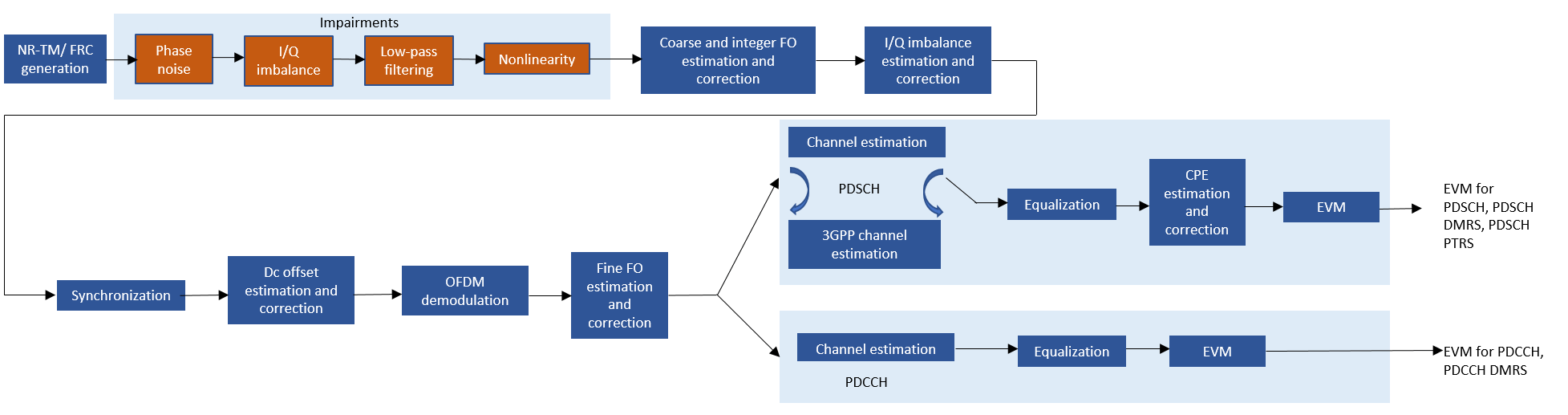To introduce I/Q imbalance, apply a 0.2 dB amplitude imbalance and a 0.5 degree phase imbalance to the waveform. You can also increase the amplitude and phase imbalances by setting amplitudeImbalance and phaseImbalance to higher values.

if IQImbalanceON amplitudeImbalance = 0.2; phaseImbalance = 0.5; rxWaveform = iqimbal(rxWaveform,amplitudeImbalance,phaseImbalance); end 

To filter the baseband waveform, use a low-pass filter. If the use of the current passband and stopband frequencies, PassbandFrequency and StopbandFrequency, results in high EVM values for a certain waveform bandwidth and OSR, use a wider filter by increasing PassbandFrequency and StopbandFrequency. To use a narrower filter, reduce PassbandFrequency and StopbandFrequency. You can also modify the passband ripple and the stopband attenuation. This figure shows the magnitude response of the low-pass filter.

if filterOn % Create low-pass filter object LPF = dsp.LowpassFilter('SampleRate',sr, ... 'FilterType','IIR', ... 'PassbandFrequency',sr/2-(sr/2*0.6), ... 'StopbandFrequency',sr/2-(sr/2*0.5), ... 'PassbandRipple',0.7, ... 'StopbandAttenuation',60); % Plot the magnitude response of the low-pass filter [h,w] = freqz(LPF); figure plot(w/pi,mag2db(abs(h))); axis('tight'); grid; title('Magnitude Response of the LPF') xlabel('Normalized Frequency (x \pi rad/sample)'); ylabel('Magnitude (dB)'); % Filter the waveform rxWaveform = LPF(rxWaveform); release(LPF); end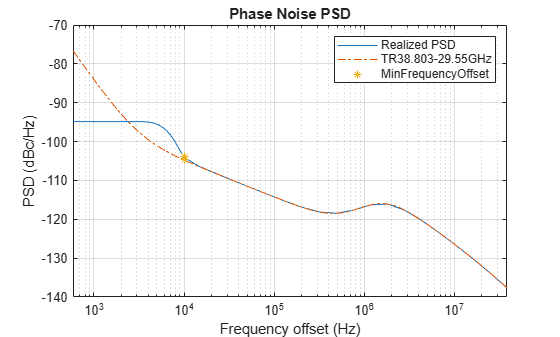For this example, use the Rapp model to introduce nonlinear distortion. This figure shows the nonlinearity introduced by the Rapp model. Set the parameters for the Rapp model to match the characteristics of the memoryless model from TR 38.803 Annex A.1.

if nonLinearityModelOn % Generate Rapp model object rapp = comm.MemorylessNonlinearity('Method','Rapp model'); rapp.Smoothness = 1.55; rapp.OutputSaturationLevel = 1; % Plot nonlinear characteristic plotNonLinearCharacteristic(rapp); % Apply nonlinearity rxWaveform = rapp(rxWaveform); release(rapp); end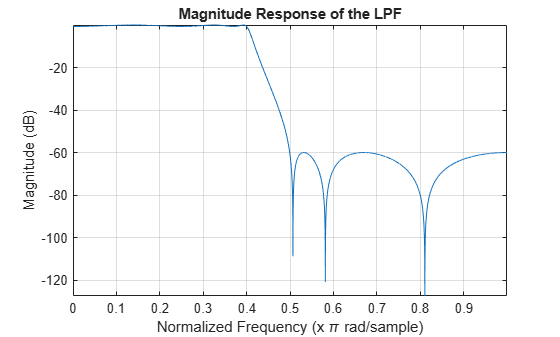Plot the spectrum of the waveform before and after adding the RF impairments

scope = spectrumAnalyzer('SampleRate',sr,... 'ChannelNames',{'Before impairments','After impairments'},... 'Title', 'Waveform before and after impairments'); scope([txWaveform,rxWaveform]); release(scope);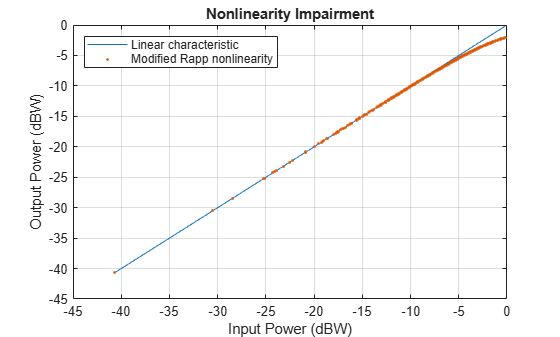The signal was previously repeated twice. Remove the first half of this signal. This avoids any transient introduced by the impairment models.

if dm == "FDD" nFrames = 1; else % TDD nFrames = 2; end rxWaveform(1:nFrames*tmwaveinfo.Info.SamplesPerSubframe*10,:) = []; 

### Measurements

The helper function hNRDownlinkEVM performs these steps to decode and analyze the waveform:

• Coarse frequency offset estimation and correction

• Integer frequency offset estimation and correction

• I/Q imbalance estimation and correction

• Synchronization using the demodulation reference signal (DM-RS) over one frame for FDD (two frames for TDD)

• Direct current (DC) offset estimation and correction

• OFDM demodulation of the received waveform

• DC subcarrier exclusion

• Fine frequency offset estimation and correction

• Channel estimation

• Equalization

• Common phase error (CPE) estimation and compensation

• PDSCH EVM computation (enable the switch evm3GPP, to process according to the EVM measurement requirements specified in TS 38.104, Annex B (FR1) / Annex C (FR2)).

• PDCCH EVM computation

The example measures and outputs various EVM related statistics per symbol, per slot, and per frame peak EVM and RMS EVM. The example displays EVM for each slot and frame. It also displays the overall EVM averaged over the entire input waveform. The example produces a number of plots: EVM vs per OFDM symbol, slot, subcarrier, and overall EVM. Each plot displays the peak vs RMS EVM.

cfg = struct(); cfg.Evm3GPP = evm3GPP; cfg.TargetRNTIs = targetRNTIs; cfg.PlotEVM = plotEVM; cfg.DisplayEVM = displayEVM; cfg.IQImbalance = IQImbalanceON; % Compute and display EVM measurements [evmInfo,eqSym,refSym] = hNRDownlinkEVM(tmwavegen.Config,rxWaveform,cfg); 
EVM stats for BWP idx : 1 PDSCH RMS EVM, Peak EVM, slot 0: 2.319 6.537% PDSCH RMS EVM, Peak EVM, slot 1: 2.428 8.852% PDSCH RMS EVM, Peak EVM, slot 2: 2.245 5.909% PDSCH RMS EVM, Peak EVM, slot 3: 2.454 7.507% PDSCH RMS EVM, Peak EVM, slot 4: 2.483 6.999% PDSCH RMS EVM, Peak EVM, slot 5: 2.585 7.484% PDSCH RMS EVM, Peak EVM, slot 6: 2.519 8.509% PDSCH RMS EVM, Peak EVM, slot 7: 2.583 8.062% PDSCH RMS EVM, Peak EVM, slot 8: 2.380 6.781% PDSCH RMS EVM, Peak EVM, slot 9: 2.774 10.522% PDSCH RMS EVM, Peak EVM, slot 10: 2.240 6.740% PDSCH RMS EVM, Peak EVM, slot 11: 2.228 7.963% PDSCH RMS EVM, Peak EVM, slot 12: 2.511 8.002% PDSCH RMS EVM, Peak EVM, slot 13: 2.459 7.831% PDSCH RMS EVM, Peak EVM, slot 14: 2.287 8.306% PDSCH RMS EVM, Peak EVM, slot 15: 2.328 8.857% PDSCH RMS EVM, Peak EVM, slot 16: 2.520 9.851% PDSCH RMS EVM, Peak EVM, slot 17: 2.412 6.223% PDSCH RMS EVM, Peak EVM, slot 18: 2.660 9.351% PDCCH RMS EVM, Peak EVM, slot 0: 2.095 4.296% PDCCH RMS EVM, Peak EVM, slot 1: 1.452 3.086% PDCCH RMS EVM, Peak EVM, slot 2: 1.813 4.080% PDCCH RMS EVM, Peak EVM, slot 3: 1.637 3.289% PDCCH RMS EVM, Peak EVM, slot 4: 1.987 3.807% PDCCH RMS EVM, Peak EVM, slot 5: 1.752 4.863% PDCCH RMS EVM, Peak EVM, slot 6: 2.375 4.750% PDCCH RMS EVM, Peak EVM, slot 7: 1.600 3.493% PDCCH RMS EVM, Peak EVM, slot 8: 1.512 3.245% PDCCH RMS EVM, Peak EVM, slot 9: 1.729 3.779% PDCCH RMS EVM, Peak EVM, slot 10: 1.384 3.092% PDCCH RMS EVM, Peak EVM, slot 11: 1.562 3.945% PDCCH RMS EVM, Peak EVM, slot 12: 1.624 3.245% PDCCH RMS EVM, Peak EVM, slot 13: 1.956 4.071% PDCCH RMS EVM, Peak EVM, slot 14: 1.740 3.993% PDCCH RMS EVM, Peak EVM, slot 15: 1.474 3.085% PDCCH RMS EVM, Peak EVM, slot 16: 1.772 3.829% PDCCH RMS EVM, Peak EVM, slot 17: 1.619 3.151% PDCCH RMS EVM, Peak EVM, slot 18: 1.867 4.254% Averaged overall PDSCH RMS EVM: 2.447% Overall PDSCH Peak EVM = 10.5224% Averaged overall PDCCH RMS EVM: 1.750% Overall PDCCH Peak EVM = 4.8629%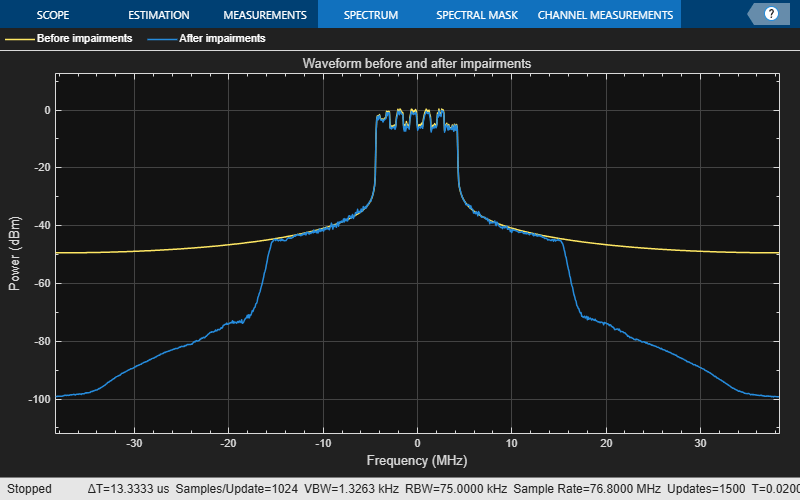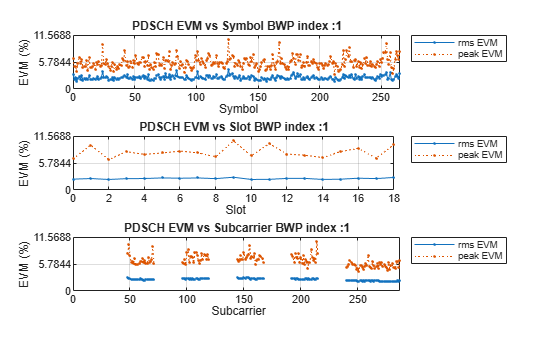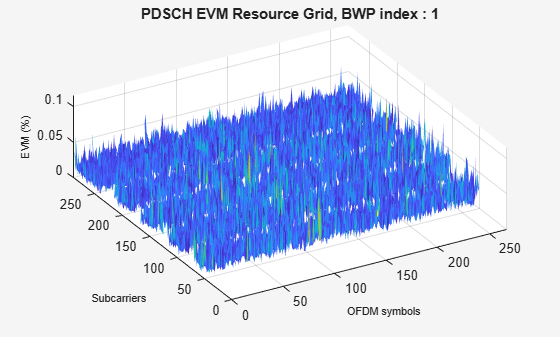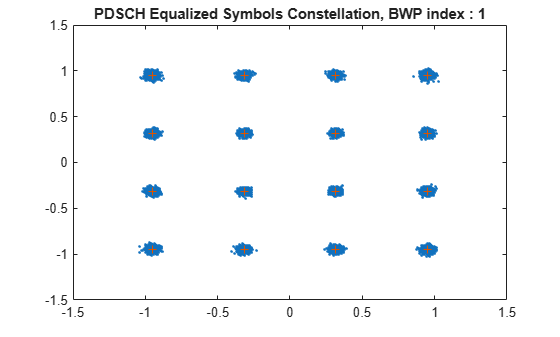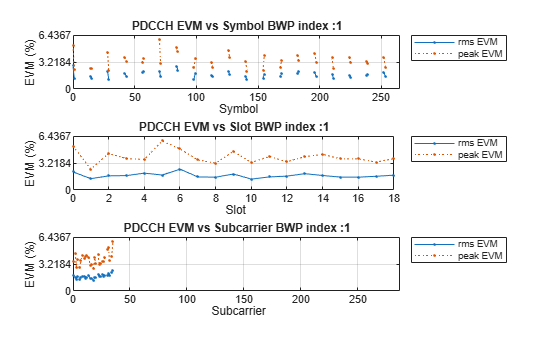### Local functions

function plotNonLinearCharacteristic(memoryLessNonlinearity) % Plot the nonlinear characteristic of the power amplifier (PA) impairment % represented by the input parameter memoryLessNonlinearity, which is a % comm.MemorylessNonlinearity Communications Toolbox(TM) System object. % Input samples x = complex((1/sqrt(2))*(-1+2*rand(1000,1)),(1/sqrt(2))*(-1+2*rand(1000,1))); % Nonlinearity yRapp = memoryLessNonlinearity(x); % Release object to feed it a different number of samples release(memoryLessNonlinearity); % Plot characteristic figure; plot(10*log10(abs(x).^2),10*log10(abs(x).^2)); hold on; grid on plot(10*log10(abs(x).^2),10*log10(abs(yRapp).^2),'.'); xlabel('Input Power (dBW)'); ylabel('Output Power (dBW)'); title('Nonlinearity Impairment') legend('Linear characteristic', 'Rapp nonlinearity','Location','Northwest'); end function [sampleRate] = getNominalSampleRate(cfgObj) % Obtain nominal sample rate for the input parameter CFGOBJ. CFGOBJ is % an object of type 'nrDLCarrierConfig' sampleRate = nr5g.internal.wavegen.maxSampleRate(cfgObj); end